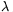Mathematical and Physical Journal
for High Schools
Issued by the MATFUND Foundation
 Already signed up? New to KöMaL?

#Problem P. 4614. (February 2014)

P. 4614. The linear charge density of a very long, straight, vertical insulating thread is=8.10-7 C/m. A very small metal ball of charge q=6.81.10-8 C is suspended by a very thin insulating thread of length=10 cm, which is at a distance of d0=5 cm from the other thread. The metal ball is fixed with an insulating equipment at the position in which the thread is vertical. At a given moment the ball is released from its fixed position without any push. Determine

a) the maximum distance between the vertical metal thread and the ball;

b) the angle between the vertical and the supporting thread of the ball, when the speed of the ball is maximum.

(5 pont)

Deadline expired on March 10, 2014.

Sorry, the solution is available only in Hungarian. Google translation

Megoldásvázlat. $\displaystyle a)$ Az $\displaystyle mg \left(\ell-\sqrt{\ell^2-(x-d_0)^2}\right)=\frac{\lambda q}{2\pi\varepsilon_0}\ln\frac{x}{d_0}$ egyenlet közelítő megoldása: $\displaystyle x=13{,}7~$cm.

$\displaystyle b)$ A $\displaystyle \frac{\lambda q}{2\pi\varepsilon_0 mg}=\tg\alpha\,(d_0+\ell\sin\alpha)$ egyenlet numerikus megoldása: $\displaystyle \alpha=27{,}5^\circ$.

### Statistics:

 29 students sent a solution. 5 points: Antalicz Balázs, Balogh Menyhért, Berta Dénes, Büki Máté, Csathó Botond, Di Giovanni Márk, Fehér Zsombor, Fekete Panna, Forrai Botond, Gróf Tamás, Holczer András, Horicsányi Attila, Juhász Péter, Kaposvári Péter, Marosvári Kristóf, Olosz Balázs, Sal Kristóf, Sárvári Péter. 4 points: Blum Balázs, Janzer Barnabás. 3 points: 2 students. 2 points: 5 students. 1 point: 2 students.

Problems in Physics of KöMaL, February 2014